# 1 Setup

This article will teach you how to use `ggpredict()` and `plot()` to visualize the marginal effects of one or more variables of interest in linear and logistic regression models. You will learn how to specify predictor values and how to fix covariates at specific values, in addition to options for customizing plots.

Marginal means are predicted outcomes given certain constraints, and a marginal effect is the predicted change in the outcome after varying a variable of interest while holding others constant.

As our models grow in complexity and dimensionality, we face increasing difficulty in interpreting coefficients. Visualizing margins helps us better understand and communicate our model results.

To begin, load the `ggeffects` and `ggplot2` libraries. `ggeffects` has the `ggpredict()` function, which we will use to calculate margins, and `ggplot2` has other plotting functions.

``````library(ggeffects)
library(ggplot2)``````

Load a sample of the 2019 American Community Survey. The variables in this dataset are described in Regression Diagnostics with R.

``acs <- readRDS(url("https://sscc.wisc.edu/sscc/pubs/data/RegDiag/acs2019sample.rds"))``

Fit a linear model predicting `income` from `age`, `education`, the interaction of the two, `hours_worked` per week, and `sex`.

``mod <- lm(income ~ age * education + hours_worked + sex, acs)``

Now, suppose we want to understand the marginal effect of `age` for females with a bachelor’s degree who work 40 hours per week.

View the model estimates with `summary()`:

``summary(mod)``
``````##
## Call:
## lm(formula = income ~ age * education + hours_worked + sex, data = acs)
##
## Residuals:
##     Min      1Q  Median      3Q     Max
## -104651  -20303   -5457   10053  575865
##
## Coefficients:
##                                  Estimate Std. Error t value Pr(>|t|)
## (Intercept)                     -14651.75    7303.23  -2.006 0.044933 *
## age                                321.88     188.29   1.709 0.087479 .
## educationHigh school             -2538.18    8806.58  -0.288 0.773204
## educationSome college           -11156.06    9145.48  -1.220 0.222629
## educationAssociate's degree       6956.78   12208.20   0.570 0.568829
## educationBachelor's degree       -2617.29   10355.82  -0.253 0.800491
## educationAdvanced degree         43542.41   15015.65   2.900 0.003764 **
## hours_worked                      1061.12      72.49  14.637  < 2e-16 ***
## sexFemale                       -15115.84    1966.42  -7.687 2.08e-14 ***
## age:educationHigh school           174.06     213.52   0.815 0.415029
## age:educationSome college          505.64     223.04   2.267 0.023466 *
## age:educationAssociate's degree    154.85     280.84   0.551 0.581415
## age:educationBachelor's degree     813.06     246.26   3.302 0.000973 ***
## age:educationAdvanced degree       329.19     316.10   1.041 0.297781
## ---
## Signif. codes:  0 '***' 0.001 '**' 0.01 '*' 0.05 '.' 0.1 ' ' 1
##
## Residual standard error: 49320 on 2747 degrees of freedom
##   (2239 observations deleted due to missingness)
## Multiple R-squared:  0.2391, Adjusted R-squared:  0.2355
## F-statistic: 66.39 on 13 and 2747 DF,  p-value: < 2.2e-16``````

Our model estimates give us the coefficient estimates and significance tests we have been trained to interpret and report, but they are less useful when we want to calculate expected outcomes.

`ggpredict()` with `plot()` provides a visual aid for select terms. The two are complementary; neither can fully replace the other.

``````ggpredict(mod,
terms = "age",
condition = c(education = "Bachelor's degree",
hours_worked = 40,
sex = "Female")) |>
plot()``````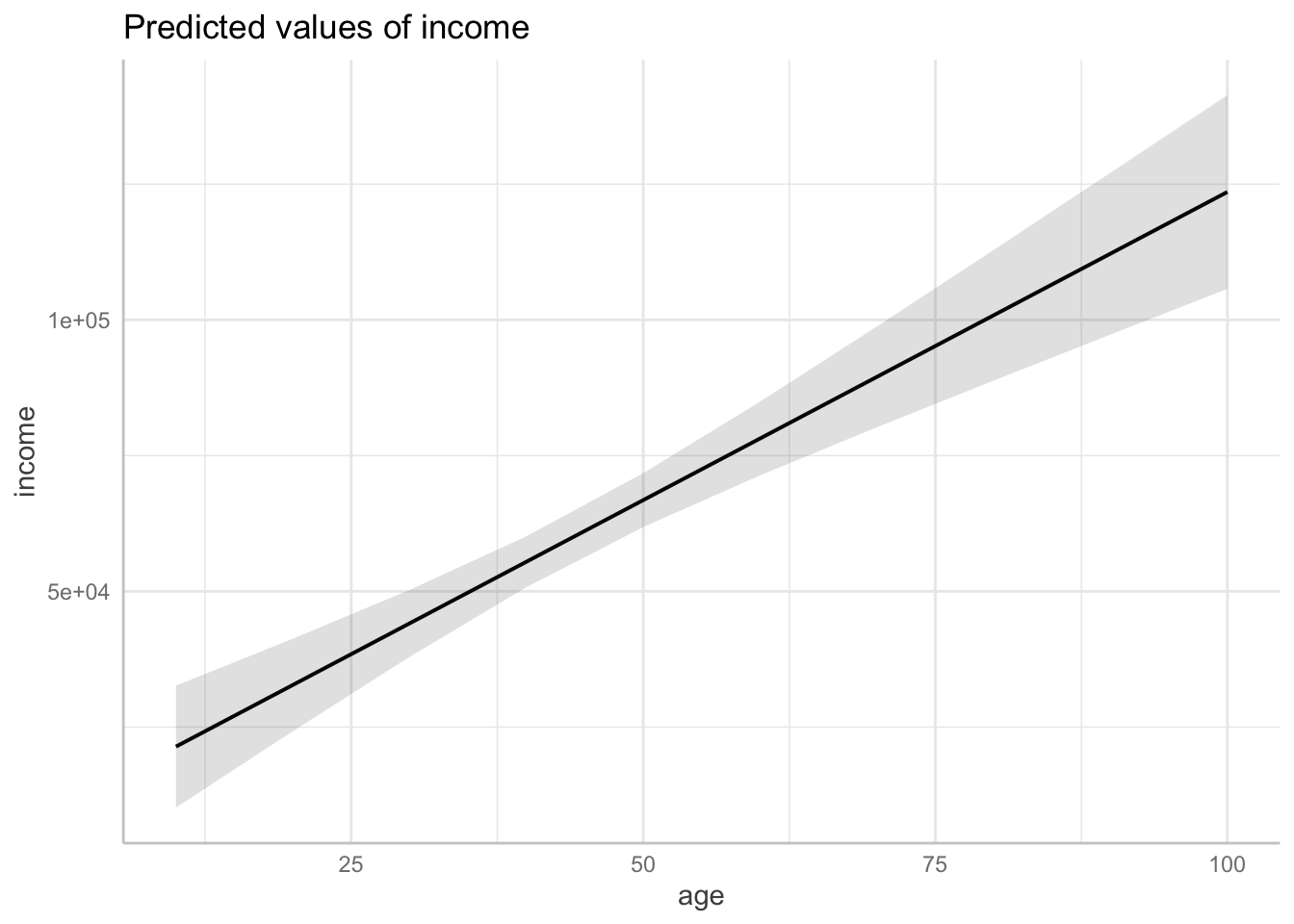This plot helps us visualize the marginal effect of age on income when we hold `education`, `hours_worked`, and `sex` at specific values. The expected increase in income with age appears to be quite substantial.

# 2 Plotting Margins

We will continue to plot margins from `mod`, our regression model fit to the `acs` dataset.

We will use two functions to create margins plots: `ggpredict()` and `plot()`. `ggeffects` has an additional method for `plot()` to create margins plots with `ggplot2`.

Two arguments of `ggpredict()` that we will use are `model` and `terms`. `model` is just the name of our fitted model, `mod`.

`terms` takes a character vector of up to four predictor names, but here we will only use three. The three terms are mapped to the ggplot aesthetics of `x`, `group`, and `facet`, in that order.

• `x` is mapped to the x-axis
• `group`s have different lines, colors, and/or shapes
• `facet`s are separate sub-plots

The three terms take different types of variables.

• `x` can be continuous or categorical
• `group` and `facet` must be categorical, or they will be made categorical
• See Margins at Specific Values to specify how continuous variables are handled (e.g., by using specific values, mean ± SD, etc.)

## 2.1 Terms

Our model has two continuous (`age`, `hours_worked`) and two categorical variables (`education`, `sex`). We can create a range of plots with different numbers and orders of these variables.

One continuous:

``ggpredict(mod, terms = c("age")) |> plot()``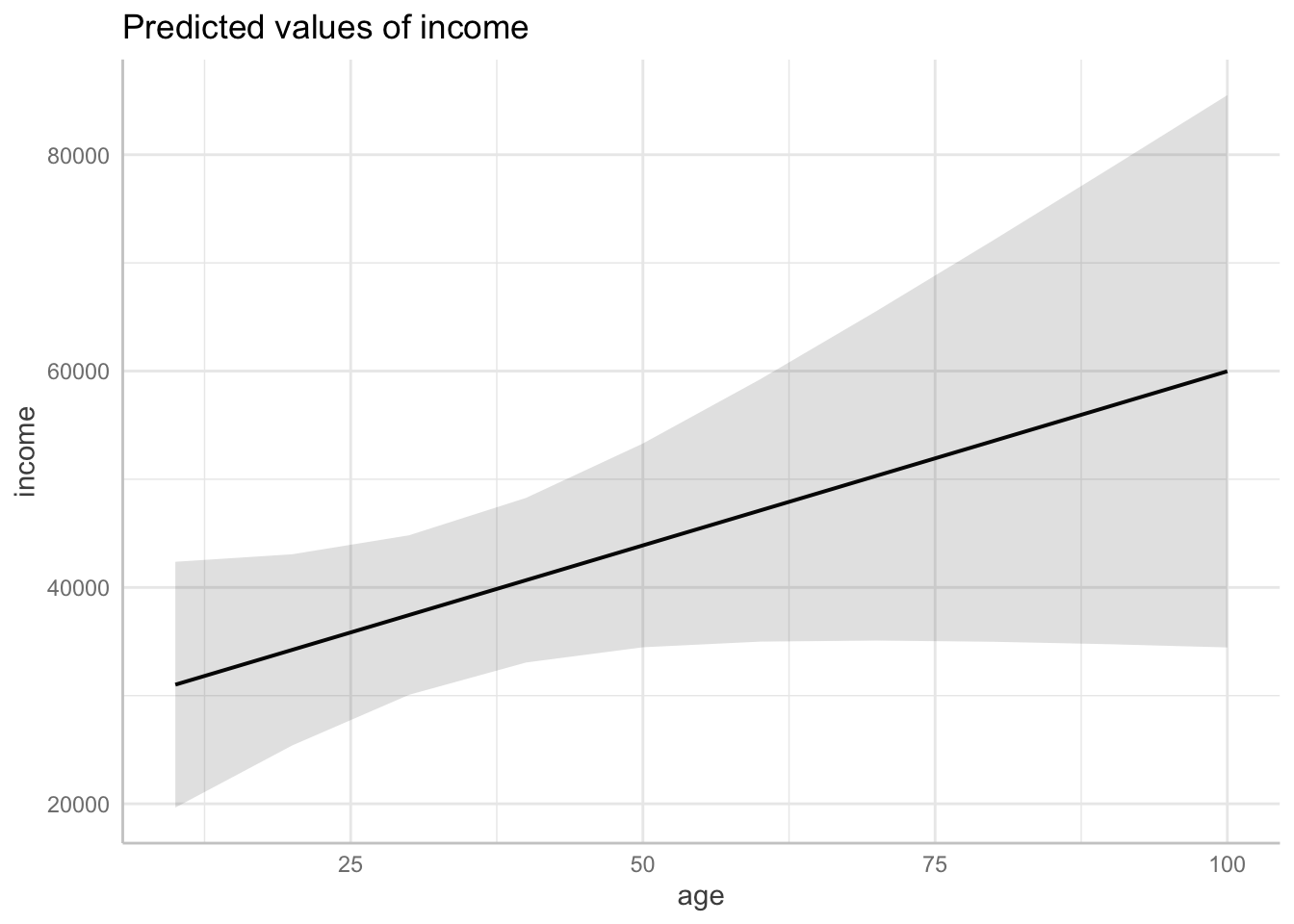One categorical:

``ggpredict(mod, terms = c("education")) |> plot()``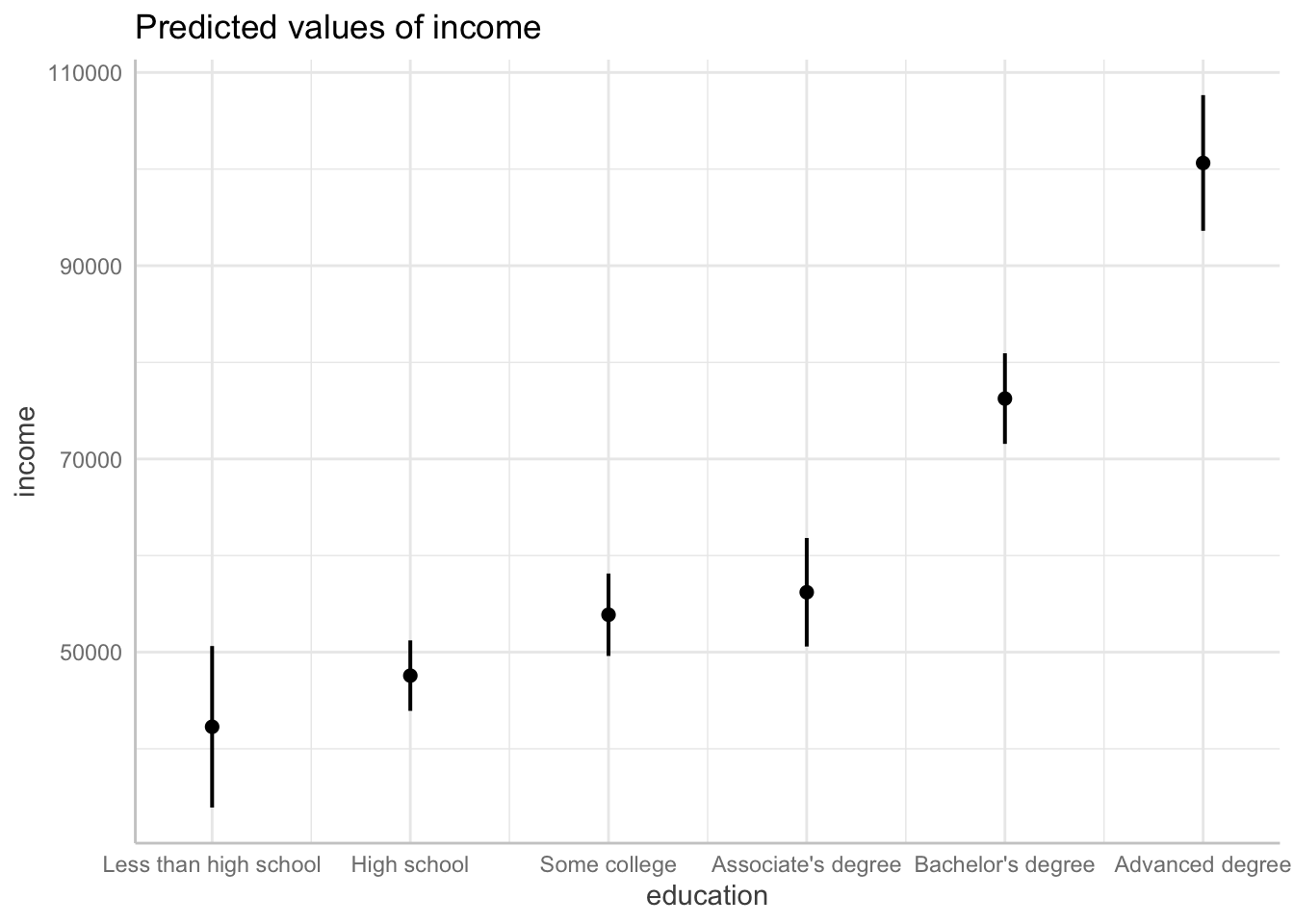Two continuous:

``ggpredict(mod, terms = c("age", "hours_worked")) |> plot()``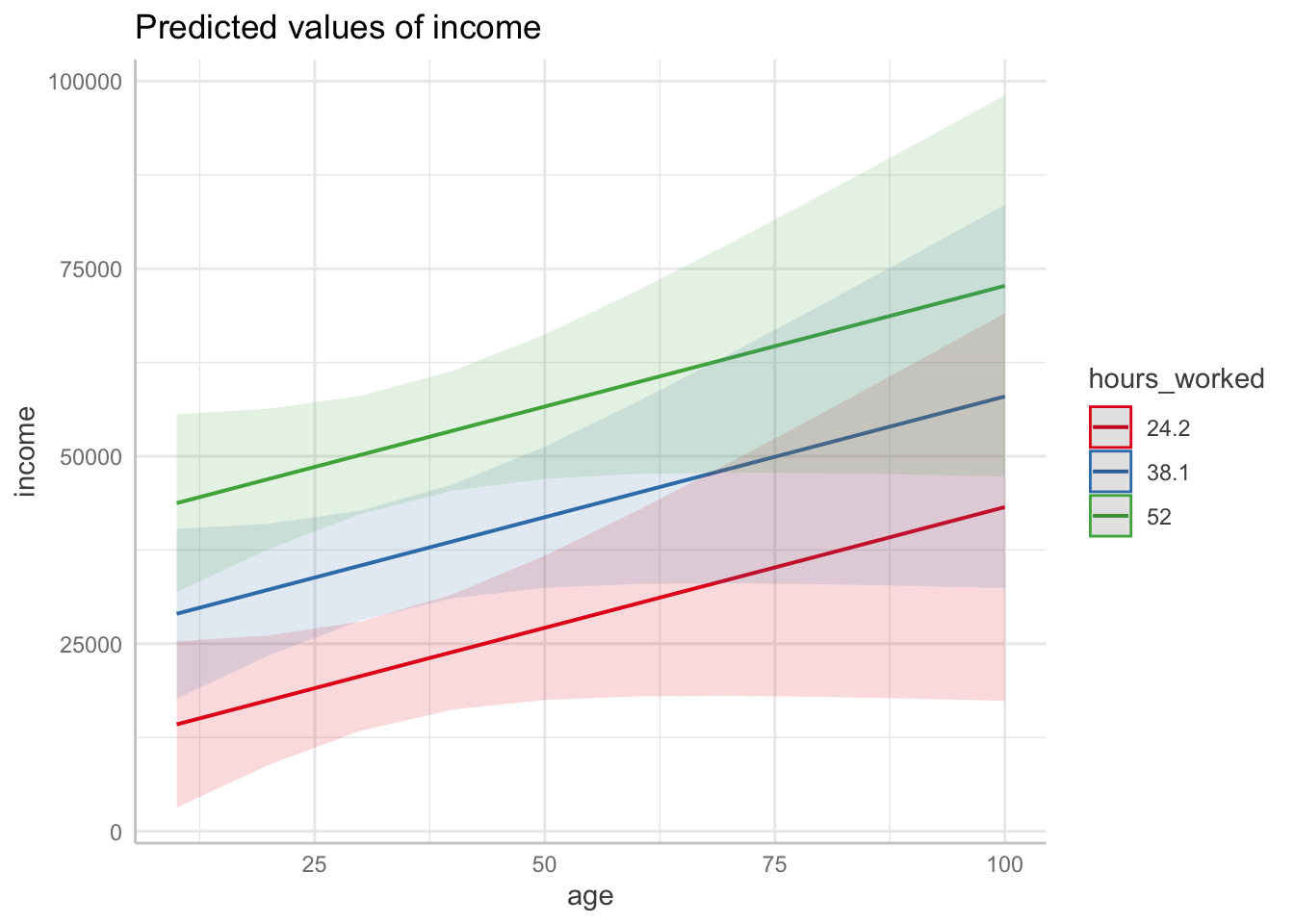Two categorical:

``ggpredict(mod, terms = c("education", "sex")) |> plot(colors = "bw")``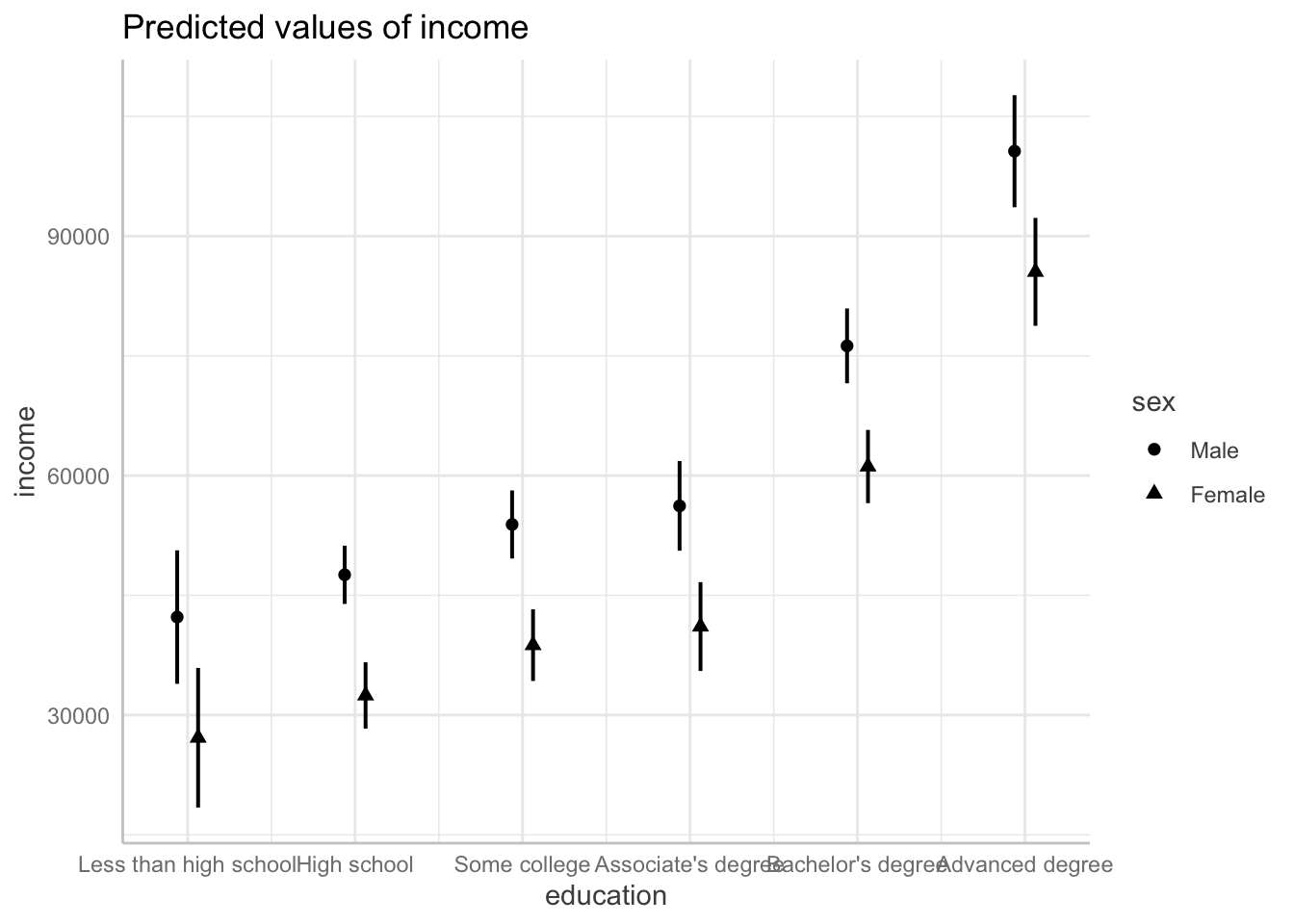One continuous then one categorical:

``ggpredict(mod, terms = c("age", "sex")) |> plot()``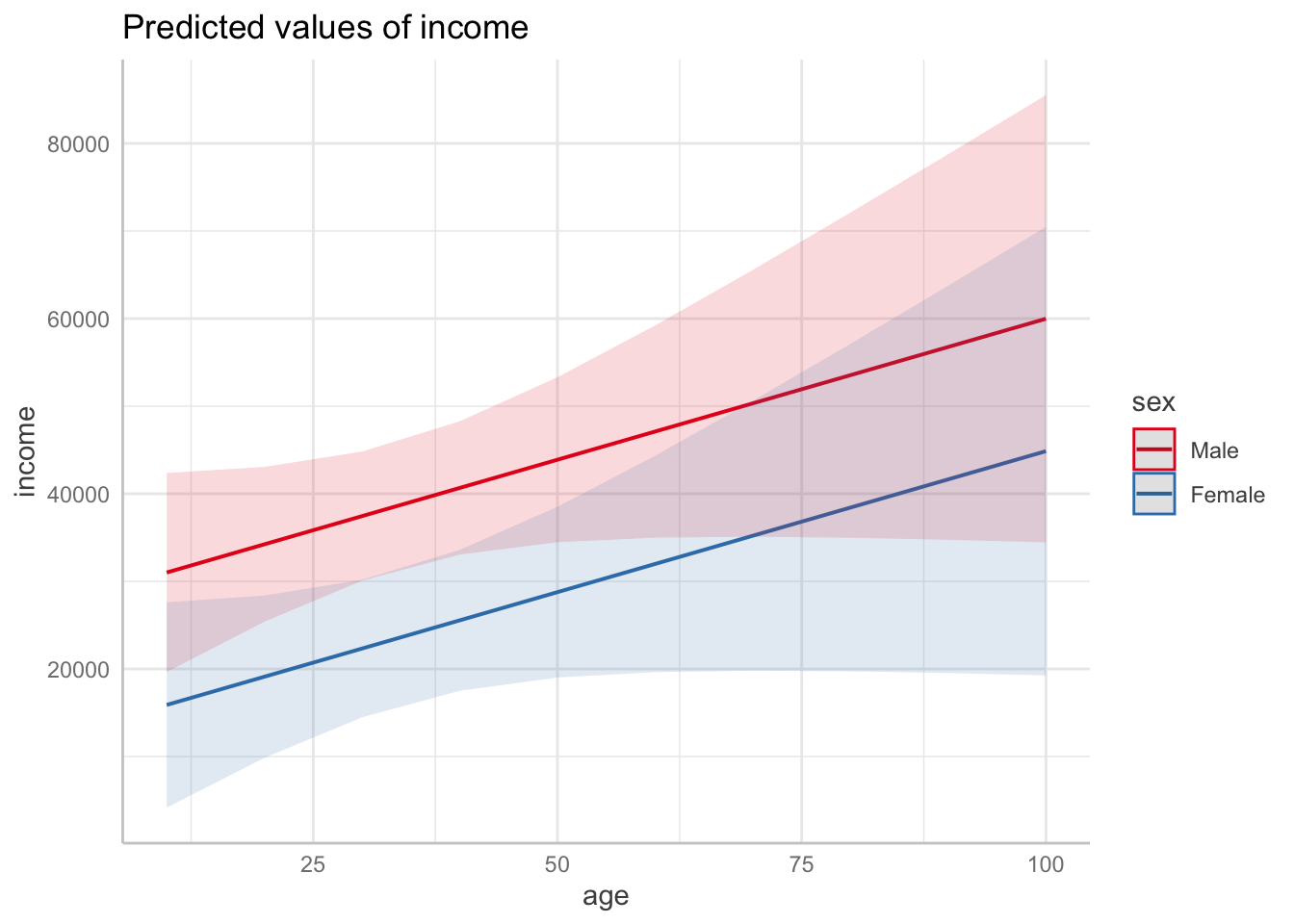One categorical then one continuous. Compare this plot to the previous plot, noticing how `age` is turned into a categorical variable since it is the `group` term.

``ggpredict(mod, terms = c("sex", "age")) |> plot()``